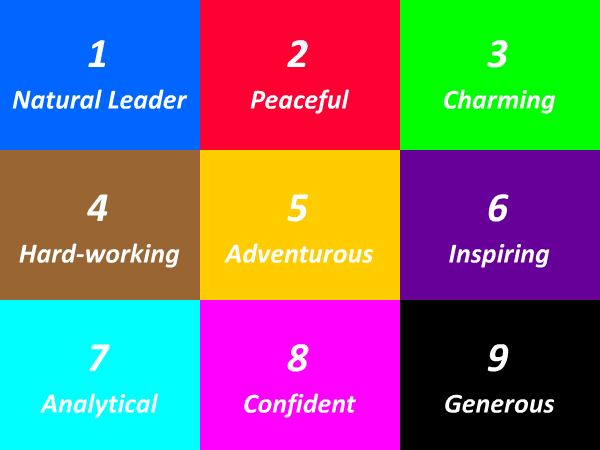# 53 Times Table - Generate Multiplication Table of 53

53 Times Table
1x53=53
2x53=106
3x53=159
4x53=212
5x53=265
6x53=318
7x53=371
8x53=424
9x53=477
10x53=530
11x53=583
12x53=636
13x53=689
14x53=742
15x53=795
16x53=848
17x53=901
18x53=954
19x53=1007
20x53=1060
21x53=1113
22x53=1166
23x53=1219
24x53=1272
25x53=1325
53 Times Table
26x53=1378
27x53=1431
28x53=1484
29x53=1537
30x53=1590
31x53=1643
32x53=1696
33x53=1749
34x53=1802
35x53=1855
36x53=1908
37x53=1961
38x53=2014
39x53=2067
40x53=2120
41x53=2173
42x53=2226
43x53=2279
44x53=2332
45x53=2385
46x53=2438
47x53=2491
48x53=2544
49x53=2597
50x53=2650
53 Times Table
51x53=2703
52x53=2756
53x53=2809
54x53=2862
55x53=2915
56x53=2968
57x53=3021
58x53=3074
59x53=3127
60x53=3180
61x53=3233
62x53=3286
63x53=3339
64x53=3392
65x53=3445
66x53=3498
67x53=3551
68x53=3604
69x53=3657
70x53=3710
71x53=3763
72x53=3816
73x53=3869
74x53=3922
75x53=3975

## Test Your Skills Here

The 53 times table quiz is very useful in the improvement of kid's multiplication and maths skills. Here you will find a selection of times table tests designed to help your child to learn and practice their times tables. Fill in your answers. Once you have entered all the answers, click on 'Check' button to see whether you have got them all correct. If your answers is Wrong then we will show you the 'Correct' answer.

Practice Excersise
53 x = 583
53 x 3 =
53 x 4 =
53 x = 1325
53 x = 106
53 x 20 =
53 x 18 =
53 x = 1325
53 x = 1431
53 x 17 =#### More Tables#### Top Search Quesries for this page:

• 53 times table
• 53 times tables
• table of 53
• 53 multiplication table
• multiplication chart 53
• multiplication of 53
• 53 table maths### Spreading Knowledge Across the World

USA - United States of America  Canada  United Kingdom  Australia  New Zealand  South America  Brazil  Portugal  Netherland  South Africa  Ethiopia  Zambia  Singapore  Malaysia  India  China  UAE - Saudi Arabia  Qatar  Oman  Kuwait  Bahrain  Dubai  Israil  England  Scotland  Norway  Ireland  Denmark  France  Spain  Poland  and  many more....